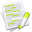#wx.GraphicsMatrix¶

A wx.GraphicsMatrix is a native representation of an affine matrix.

The contents are specific and private to the respective renderer. Instances are ref counted and can therefore be assigned as usual. The only way to get a valid instance is via wx.GraphicsContext.CreateMatrix or wx.GraphicsRenderer.CreateMatrix .

##Class Hierarchy¶Inheritance diagram for class GraphicsMatrix:

##Methods Summary¶

 Concat Concatenates the matrix passed with the current matrix. Get Returns the component values of the matrix via the argument pointers. GetNativeMatrix Returns the native representation of the matrix. Invert Inverts the matrix. IsEqual Returns True if the elements of the transformation matrix are equal. IsIdentity Return True if this is the identity matrix. Rotate Rotates this matrix clockwise (in radians). Scale Scales this matrix. Set Sets the matrix to the respective values (default values are the identity matrix). TransformDistance Applies this matrix to a distance (ie. TransformPoint Applies this matrix to a point. Translate Translates this matrix.

##Class API¶

class wx.GraphicsMatrix(GraphicsObject)

A GraphicsMatrix is a native representation of an affine matrix.

### Methods¶

Concat(self, t)

Concatenates the matrix passed with the current matrix.

The effect of the resulting transformation is to first apply the transformation in t to the coordinates and then apply the transformation in the current matrix to the coordinates.

# matrix = t * matrix

Parameters

t (wx.GraphicsMatrix) – The parameter matrix is the multiplicand.

Get(self)

Returns the component values of the matrix via the argument pointers.

Return type

tuple

Returns

( a, b, c, d, tx, ty )

GetNativeMatrix(self)

Returns the native representation of the matrix.

For CoreGraphics this is a CFAffineMatrix pointer, for GDIPlus a Matrix Pointer, and for Cairo a cairo_matrix_t pointer.

Invert(self)

Inverts the matrix.

IsEqual(self, t)

Returns True if the elements of the transformation matrix are equal.

Parameters

t (wx.GraphicsMatrix) –

Return type

bool

IsIdentity(self)

Return True if this is the identity matrix.

Return type

bool

Rotate(self, angle)

Rotates this matrix clockwise (in radians).

Parameters

angle (wx.Double) – Rotation angle in radians, clockwise.

Scale(self, xScale, yScale)

Scales this matrix.

Parameters
• xScale (wx.Double) –

• yScale (wx.Double) –

Set(self, a=1.0, b=0.0, c=0.0, d=1.0, tx=0.0, ty=0.0)

Sets the matrix to the respective values (default values are the identity matrix).

Parameters
• a (wx.Double) –

• b (wx.Double) –

• c (wx.Double) –

• d (wx.Double) –

• tx (wx.Double) –

• ty (wx.Double) –

TransformDistance(self, dx, dy)

Applies this matrix to a distance (ie.

performs all transforms except translations).

Parameters
• dx (wx.Double) –

• dy (wx.Double) –

Return type

tuple

Returns

( dx, dy )

TransformPoint(self, x, y)

Applies this matrix to a point.

Parameters
• x (wx.Double) –

• y (wx.Double) –

Return type

tuple

Returns

( x, y )

Translate(self, dx, dy)

Translates this matrix.

Parameters
• dx (wx.Double) –

• dy (wx.Double) –

### Properties¶

NativeMatrix